Function Repository Resource:

# ContrapedalCurve

Compute the contrapedal curve

Contributed by: Alfred Gray
 ResourceFunction["ContrapedalCurve"][{a,b},c,t] gives the contrapedal curve of the plane curve c with respect to the point {a,b} and parameter t.

## Details and Options

The contrapedal curve is the analog of the pedal curve but computed using the normal instead of the tangent.
The pedal of a given curve c from a fixed point p is the locus of the foot of the perpendicular from p to the tangent to c.
The contrapedal curve (or normal pedal curve) is the pedal curve of the evolute of a curve c.

## Examples

### Basic Examples (3)

Define a cardioid:

 In:=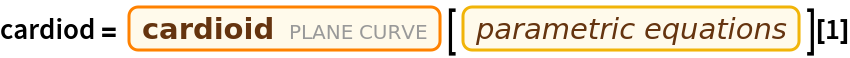Out=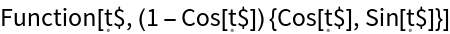Compute the contrapedal of the cardioid:

 In:=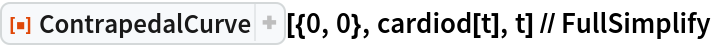Out=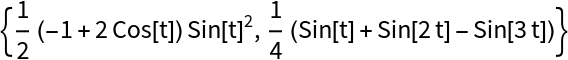Plot the cardioid along with its contrapedal:

 In:=Out=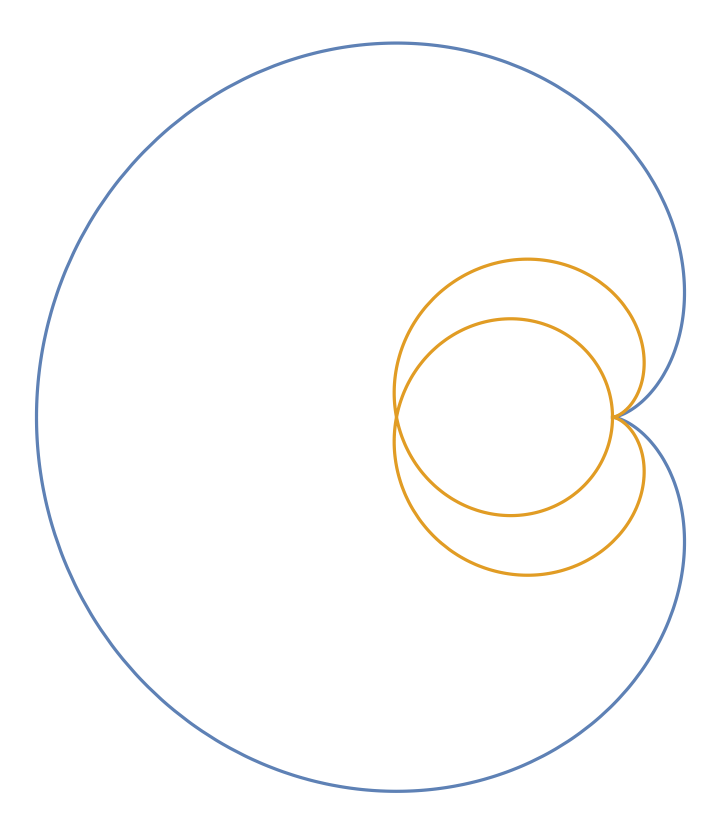### Basic Examples (3)

Define an ellipse:

 In:=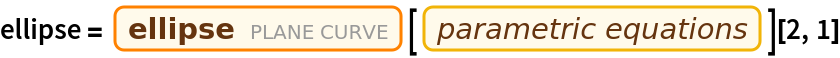Out=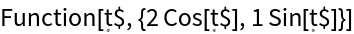Compute the contrapedal of the ellipse:

 In:=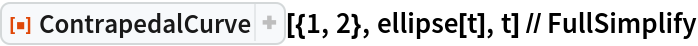Out=Interactively plot the ellipse along with its contrapedal curve, varying the base point:

 In:=Out=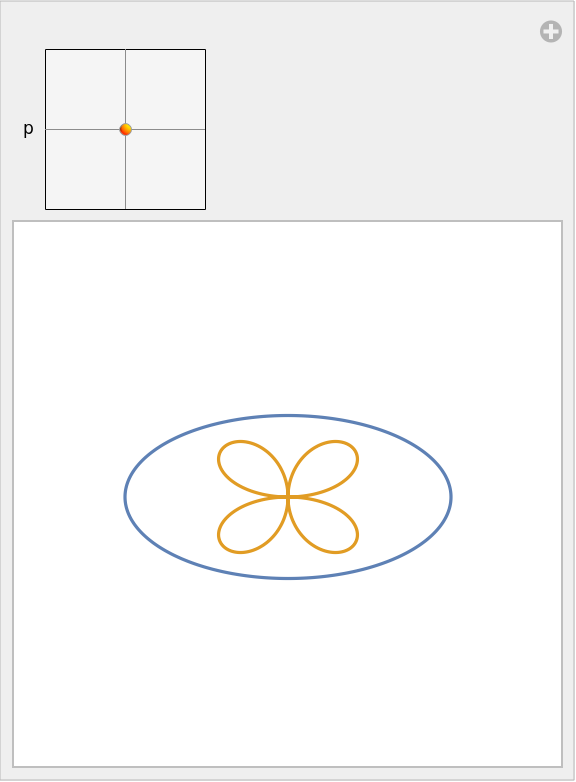Enrique Zeleny

## Version History

• 1.0.0 – 26 February 2020